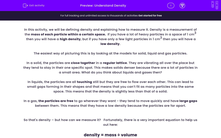# Understand Density

In this worksheet, students will learn how to measure density and use the density equation.Key stage:  KS 4

Year:  GCSE

GCSE Boards:   AQA, AQA Trilogy, AQA Synergy, OCR 21st Century, OCR Gateway, Pearson Edexcel, Eduqas,

Popular topics:   Physics worksheets

Difficulty level:#### Worksheet Overview

In this activity, we will be defining density and explaining how to measure it. Density is a measurement of the mass of each particle within a certain space.  If you have a lot of heavy particles in a space of 1 cm3  then you will have a high density, but if you have only a few light particles in 1 cm3 then you will have a low density.

The easiest way of picturing this is by looking at the models for solid, liquid and gas particles.

In a solid, the particles are close together in a regular lattice. They are vibrating all over the place but they tend to stay in their one specific spot. This makes solids denser because there are a lot of particles in a small area. What do you think about liquids and gases then?

In liquids, the particles are all touching still but they are free to flow over each other. This can lead to small gaps forming in their shapes and that means that you can’t fit as many particles into the same space. This means that the density is slightly less than that of a solid.

In a gas, the particles are free to go wherever they want - they tend to move quickly and have large gaps between them. This means that they have a low density because the particles are far apart.

So that's density – but how can we measure it?   Fortunately, there is a very important equation to help us out here:

density = mass ÷ volume

ρ = m ÷ V

That funny sign (ρ) is the Greek letter rho which, in this case, means density. Density is measured in kg/m3  (kilograms per metre cubed), mass is measured in kg (kilograms) and volume is measured in m3 (metres cubed).

Let's have a look at how we can use this equation to find the density of an object. By looking at the equation, we know that in order to work this out, we have to know its mass and volume.

Working out the mass is simple - you just need to put the object on a top pan balance and read off the measurement that it gives you (making sure that you zero the balance to start off with). Let's say that the object weighs 0.5 kg.

The volume isn’t all that difficult either -  all you need to do is measure the length, width, and depth of the object. You then multiply these numbers together and you get your volume. Let's say your object measures 0.25 m x 0.25 m x 0.3 m =   0.018 m3

So, put this into the equation:

ρ = m ÷ V

density = 0.5 ÷  0.018

density = 27.77 kg/m3

DON’T FORGET the units! Always put them in unless the question tells you otherwise!

And that’s it! Now you are a master of density! Let’s put your newfound knowledge to the test!

### What is EdPlace?

We're your National Curriculum aligned online education content provider helping each child succeed in English, maths and science from year 1 to GCSE. With an EdPlace account you’ll be able to track and measure progress, helping each child achieve their best. We build confidence and attainment by personalising each child’s learning at a level that suits them.

Get started